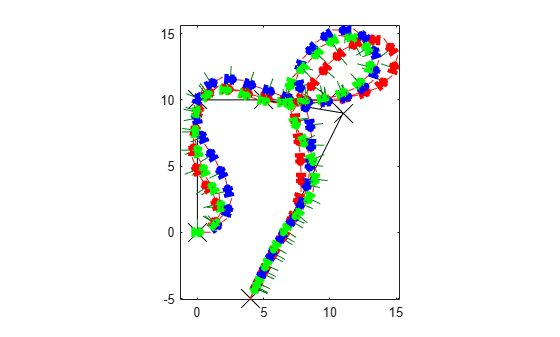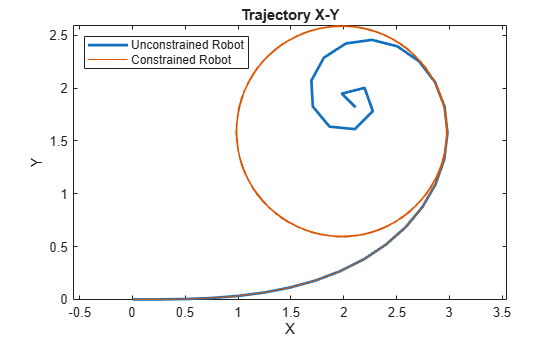# derivative

Time derivative of vehicle state

## Syntax

``stateDot = derivative(motionModel,state,cmds)``

## Description

example

````stateDot = derivative(motionModel,state,cmds)` returns the current state derivative, `stateDot`, as a three-element vector [xDot yDot thetaDot] if the motion model is a `bicycleKinematics`, `differentialDriveKinematics`, or `unicycleKinematics` object. It returns `state` as a four-element vector, [xDot yDot thetaDot psiDot], if the motion model is a `ackermannKinematics` object. xDot and yDot refer to the vehicle velocity, specified in meters per second. thetaDot is the angular velocity of the vehicle heading and psiDot is the angular velocity of the vehicle steering, both specified in radians per second.```

## Examples

collapse all

This example shows how to model different robot kinematics models in an environment and compare them.

Define Mobile Robots with Kinematic Constraints

There are a number of ways to model the kinematics of mobile robots. All dictate how the wheel velocities are related to the robot state: `[x y theta]`, as xy-coordinates and a robot heading, `theta`, in radians.

Unicycle Kinematic Model

The simplest way to represent mobile robot vehicle kinematics is with a unicycle model, which has a wheel speed set by a rotation about a central axle, and can pivot about its z-axis. Both the differential-drive and bicycle kinematic models reduce down to unicycle kinematics when inputs are provided as vehicle speed and vehicle heading rate and other constraints are not considered.

`unicycle = unicycleKinematics("VehicleInputs","VehicleSpeedHeadingRate");`

Differential-Drive Kinematic Model

The differential drive model uses a rear driving axle to control both vehicle speed and head rate. The wheels on the driving axle can spin in both directions. Since most mobile robots have some interface to the low-level wheel commands, this model will again use vehicle speed and heading rate as input to simplify the vehicle control.

`diffDrive = differentialDriveKinematics("VehicleInputs","VehicleSpeedHeadingRate");`

To differentiate the behavior from the unicycle model, add a wheel speed velocity constraint to the differential-drive kinematic model

`diffDrive.WheelSpeedRange = [-10 10]*2*pi;`

Bicycle Kinematic Model

The bicycle model treats the robot as a car-like model with two axles: a rear driving axle, and a front axle that turns about the z-axis. The bicycle model works under the assumption that wheels on each axle can be modeled as a single, centered wheel, and that the front wheel heading can be directly set, like a bicycle.

`bicycle = bicycleKinematics("VehicleInputs","VehicleSpeedHeadingRate","MaxSteeringAngle",pi/8);`

Other Models

The Ackermann kinematic model is a modified car-like model that assumes Ackermann steering. In most car-like vehicles, the front wheels do not turn about the same axis, but instead turn on slightly different axes to ensure that they ride on concentric circles about the center of the vehicle's turn. This difference in turning angle is called Ackermann steering, and is typically enforced by a mechanism in actual vehicles. From a vehicle and wheel kinematics standpoint, it can be enforced by treating the steering angle as a rate input.

`carLike = ackermannKinematics;`

Set up Simulation Parameters

These mobile robots will follow a set of waypoints that is designed to show some differences caused by differing kinematics.

```waypoints = [0 0; 0 10; 10 10; 5 10; 11 9; 4 -5]; % Define the total time and the sample rate sampleTime = 0.05; % Sample time [s] tVec = 0:sampleTime:20; % Time array initPose = [waypoints(1,:)'; 0]; % Initial pose (x y theta)```

Create a Vehicle Controller

The vehicles follow a set of waypoints using a Pure Pursuit controller. Given a set of waypoints, the robot current state, and some other parameters, the controller outputs vehicle speed and heading rate.

```% Define a controller. Each robot requires its own controller controller1 = controllerPurePursuit("Waypoints",waypoints,"DesiredLinearVelocity",3,"MaxAngularVelocity",3*pi); controller2 = controllerPurePursuit("Waypoints",waypoints,"DesiredLinearVelocity",3,"MaxAngularVelocity",3*pi); controller3 = controllerPurePursuit("Waypoints",waypoints,"DesiredLinearVelocity",3,"MaxAngularVelocity",3*pi);```

Simulate the Models Using an ODE Solver

The models are simulated using the `derivative` function to update the state. This example uses an ordinary differential equation (ODE) solver to generate a solution. Another way would be to update the state using a loop, as shown in Path Following for a Differential Drive Robot.

Since the ODE solver requires all outputs to be provided as a single output, the pure pursuit controller must be wrapped in a function that outputs the linear velocity and heading angular velocity as a single output. An example helper, `exampleHelperMobileRobotController`, is used for that purpose. The example helper also ensures that the robot stops when it is within a specified radius of the goal.

```goalPoints = waypoints(end,:)'; goalRadius = 1;```

`ode45` is called once for each type of model. The derivative function computes the state outputs with initial state set by `initPose`. Each derivative accepts the corresponding kinematic model object, the current robot pose, and the output of the controller at that pose.

```% Compute trajectories for each kinematic model under motion control [tUnicycle,unicyclePose] = ode45(@(t,y)derivative(unicycle,y,exampleHelperMobileRobotController(controller1,y,goalPoints,goalRadius)),tVec,initPose); [tBicycle,bicyclePose] = ode45(@(t,y)derivative(bicycle,y,exampleHelperMobileRobotController(controller2,y,goalPoints,goalRadius)),tVec,initPose); [tDiffDrive,diffDrivePose] = ode45(@(t,y)derivative(diffDrive,y,exampleHelperMobileRobotController(controller3,y,goalPoints,goalRadius)),tVec,initPose);```

Plot Results

The results of the ODE solver can be easily viewed on a single plot using `plotTransforms` to visualize the results of all trajectories at once.

The pose outputs must first be converted to indexed matrices of translations and quaternions.

```unicycleTranslations = [unicyclePose(:,1:2) zeros(length(unicyclePose),1)]; unicycleRot = axang2quat([repmat([0 0 1],length(unicyclePose),1) unicyclePose(:,3)]); bicycleTranslations = [bicyclePose(:,1:2) zeros(length(bicyclePose),1)]; bicycleRot = axang2quat([repmat([0 0 1],length(bicyclePose),1) bicyclePose(:,3)]); diffDriveTranslations = [diffDrivePose(:,1:2) zeros(length(diffDrivePose),1)]; diffDriveRot = axang2quat([repmat([0 0 1],length(diffDrivePose),1) diffDrivePose(:,3)]);```

Next, the set of all transforms can be plotted and viewed from the top. The paths of the unicycle, bicycle, and differential-drive robots are red, blue, and green, respectively. To simplify the plot, only show every tenth output.

```figure plot(waypoints(:,1),waypoints(:,2),"kx-","MarkerSize",20); hold all plotTransforms(unicycleTranslations(1:10:end,:),unicycleRot(1:10:end,:),'MeshFilePath','groundvehicle.stl',"MeshColor","r"); plotTransforms(bicycleTranslations(1:10:end,:),bicycleRot(1:10:end,:),'MeshFilePath','groundvehicle.stl',"MeshColor","b"); plotTransforms(diffDriveTranslations(1:10:end,:),diffDriveRot(1:10:end,:),'MeshFilePath','groundvehicle.stl',"MeshColor","g"); view(0,90)```Simulate a mobile robot model that uses Ackermann steering with constraints on its steering angle. During simulation, the model maintains maximum steering angle after it reaches the steering limit. To see the effect of steering saturation, you compare the trajectory of two robots, one with the constraints on the steering angle and the other without any steering constraints.

Define the Model

Define the Ackermann kinematic model. In this car-like model, the front wheels are a given distance apart. To ensure that they turn on concentric circles, the wheels have different steering angles. While turning, the front wheels receive the steering input as rate of change of steering angle.

`carLike = ackermannKinematics; `

Set Up Simulation Parameters

Set the mobile robot to follow a constant linear velocity and receive a constant steering rate as input. Simulate the constrained robot for a longer period to demonstrate steering saturation.

```velo = 5; % Constant linear velocity psidot = 1; % Constant left steering rate % Define the total time and sample rate sampleTime = 0.05; % Sample time [s] timeEnd1 = 1.5; % Simulation end time for unconstrained robot timeEnd2 = 10; % Simulation end time for constrained robot tVec1 = 0:sampleTime:timeEnd1; % Time array for unconstrained robot tVec2 = 0:sampleTime:timeEnd2; % Time array for constrained robot initPose = [0;0;0;0]; % Initial pose (x y theta phi) ```

Create Options Structure for ODE Solver

In this example, you pass an `options` structure as argument to the ODE solver. The `options` structure contains the information about the steering angle limit. To create the `options `structure, use the `Events` option of `odeset` and the created event function, `detectSteeringSaturation`. `detectSteeringSaturation` sets the maximum steering angle to 45 degrees.

For a description of how to define `detectSteeringSaturation`, see Define Event Function at the end of this example. ` `

`options = odeset('Events',@detectSteeringSaturation);`

Simulate Model Using ODE Solver

Next, you use the `derivative` function and an ODE solver, `ode45`, to solve the model and generate the solution.

```% Simulate the unconstrained robot [t1,pose1] = ode45(@(t,y)derivative(carLike,y,[velo psidot]),tVec1,initPose); % Simulate the constrained robot [t2,pose2,te,ye,ie] = ode45(@(t,y)derivative(carLike,y,[velo psidot]),tVec2,initPose,options);```

Detect Steering Saturation

When the model reaches the steering limit, it registers a timestamp of the event. The time it took to reach the limit is stored in `te`.

```if te < timeEnd2 str1 = "Steering angle limit was reached at "; str2 = " seconds"; comp = str1 + te + str2; disp(comp) end ```
```Steering angle limit was reached at 0.785 seconds ```

Simulate Constrained Robot with New Initial Conditions

Now use the state of the constrained robot before termination of integration as initial condition for the second simulation. Modify the input vector to represent steering saturation, that is, set the steering rate to zero.

```saturatedPsiDot = 0; % Steering rate after saturation cmds = [velo saturatedPsiDot]; % Command vector tVec3 = te:sampleTime:timeEnd2; % Time vector pose3 = pose2(length(pose2),:); [t3,pose3,te3,ye3,ie3] = ode45(@(t,y)derivative(carLike,y,cmds), tVec3,pose3, options);```

Plot the Results

Plot the trajectory of the robot using `plot` and the data stored in `pose. `

```figure(1) plot(pose1(:,1),pose1(:,2),'--r','LineWidth',2); hold on; plot([pose2(:,1); pose3(:,1)],[pose2(:,2);pose3(:,2)],'g'); title('Trajectory X-Y') xlabel('X') ylabel('Y') legend('Unconstrained robot','Constrained Robot','Location','northwest') axis equal```The unconstrained robot follows a spiral trajectory with decreasing radius of curvature while the constrained robot follows a circular trajectory with constant radius of curvature after the steering limit is reached.

Define Event Function

Set the event function such that integration terminates when 4th state, theta, is equal to maximum steering angle.

```function [state,isterminal,direction] = detectSteeringSaturation(t,y) maxSteerAngle = 0.785; % Maximum steering angle (pi/4 radians) state(4) = (y(4) - maxSteerAngle); % Saturation event occurs when the 4th state, theta, is equal to the max steering angle isterminal(4) = 1; % Integration is terminated when event occurs direction(4) = 0; % Bidirectional termination end```

## Input Arguments

collapse all

The mobile kinematics model object, which defines the properties of the motion model, specified as an `ackermannKinematics`, `bicycleKinematics`, `differentialDriveKinematics`, or a `unicycleKinematics` object.

Current vehicle state returned as a three-element or four-element vector, depending on the `motionModel` input:

x and y refer to the vehicle position, specified in meters per second. theta is the vehicle heading and psi is the vehicle steering angle, both specified in radians per second.

Input commands to the motion model, specified as a two-element vector that depends on the motion model.

For `ackermannKinematics` objects, the commands are ```[v psiDot]```.

For other motion models, the `VehicleInputs` property of `motionModel` determines the command vector:

• `"VehicleSpeedSteeringAngle"` –– ```[v psiDot]```

• `"VehicleSpeedHeadingRate" `–– ```[v omegaDot]```

• `"WheelSpeedHeadingRate"` (`unicycleKinematics` only) –– ```[wheelSpeed omegaDot]```

• `"WheelSpeeds"` (`differentialDriveKinematics` only) –– ```[wheelL wheelR]```

v is the vehicle velocity in the direction of motion in meters per second. psiDot is the steering angle rate in radians per second. omegaDot is the angular velocity at the rear axle. wwheelL and wheelR are the left and right wheel speeds respectively.

## Output Arguments

collapse all

The current state derivative returned as a three-element or four-element vector, depending on the `motionModel` input:

xDot and yDot refer to the vehicle velocity, specified in meters per second. thetaDot is the angular velocity of the vehicle heading and psiDot is the angular velocity of the vehicle steering, both specified in radians per second.

 Lynch, Kevin M., and Frank C. Park. Modern Robotics: Mechanics, Planning, and Control. 1st ed. Cambridge, MA: Cambridge University Press, 2017.